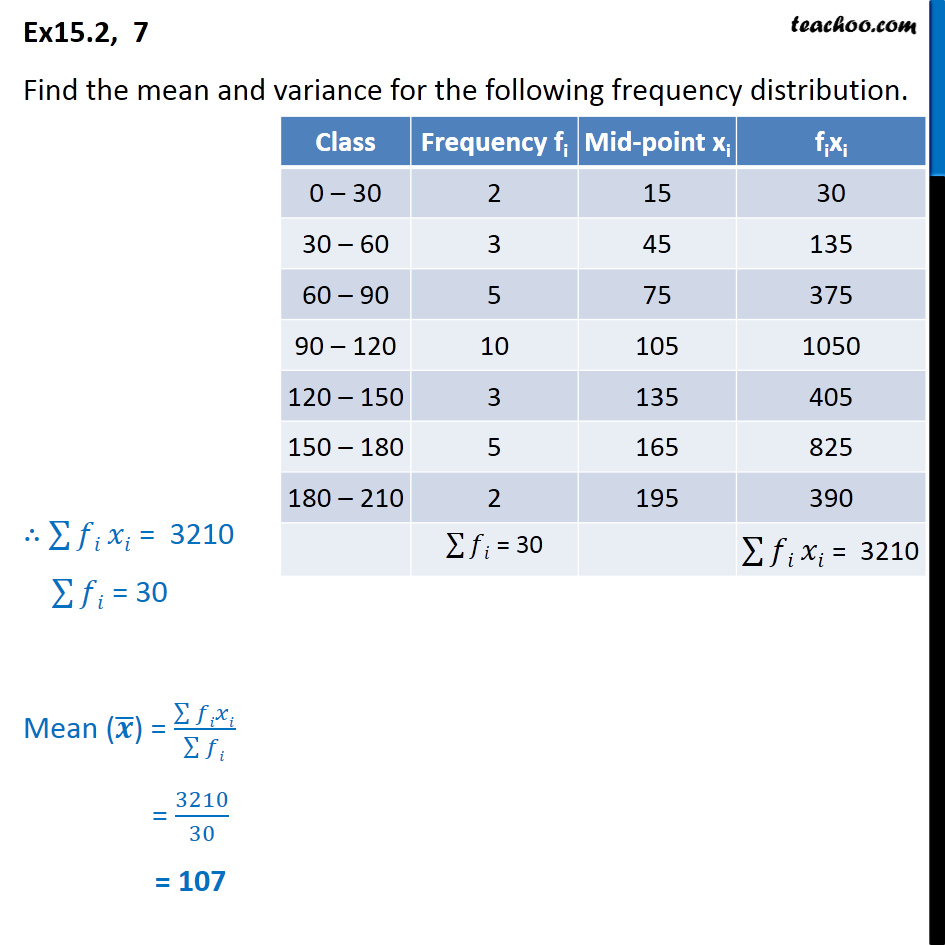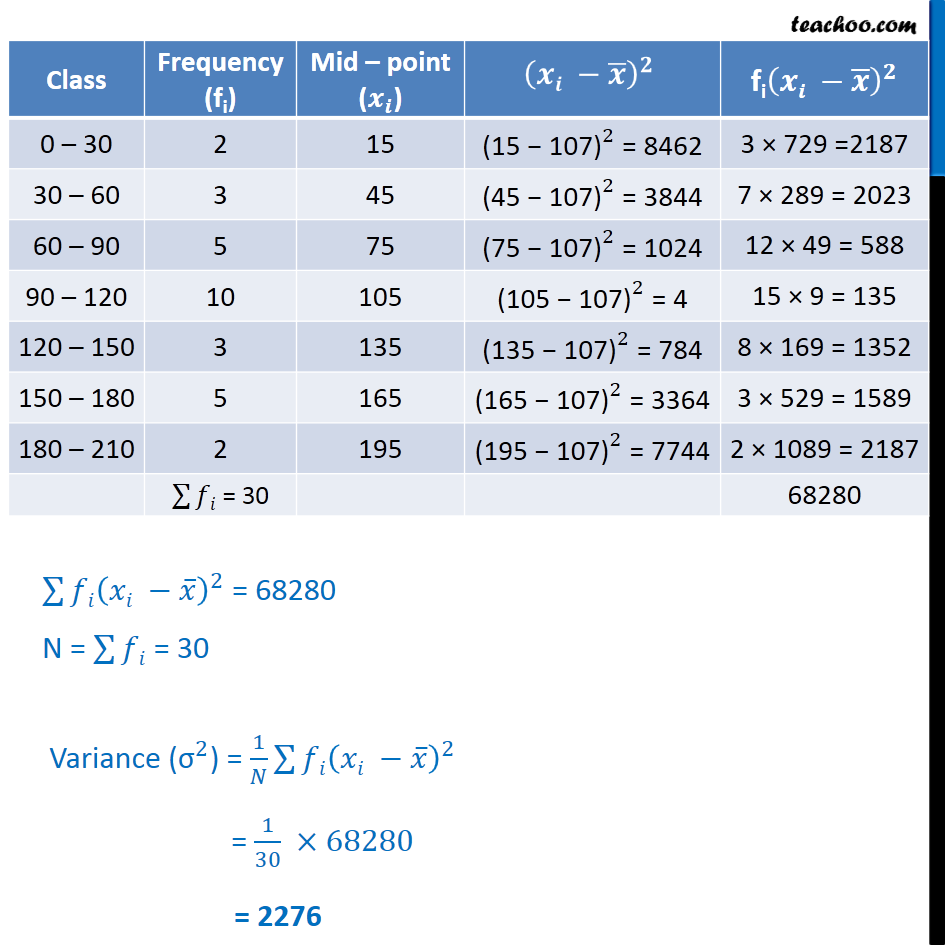Ex 15.2

Chapter 15 Class 11 Statistics (Term 1)
Serial order wise### Transcript

Ex15.2, 7 Find the mean and variance for the following frequency distribution. ( )^2 = 68280 N = = 30 Variance (" " ^2) = 1/ ( )^2 = 1/30 68280 = 2276